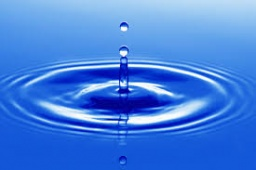# Temperature 6373

How many liters of water 80 ° C warm do we have to mix with water at a temperature of 10 ° C to get 10 liters of water 45 ° C warm?

a =  5
b =  5

### Step-by-step explanation:

80a+10b = 10·45
a+b = 10

80·a+10·b = 10·45
a+b = 10

80a+10b = 450
a+b = 10

Row 2 - 1/80 · Row 1 → Row 2
80a+10b = 450
0.88b = 4.38

b = 4.375/0.875 = 5
a = 450-10b/80 = 450-10 · 5/80 = 5

a = 5
b = 5

Our linear equations calculator calculates it.Did you find an error or inaccuracy? Feel free to write us. Thank you!

Tips for related online calculators
Do you have a linear equation or system of equations and looking for its solution? Or do you have a quadratic equation?